# Decidability Decidable Languages Chuck Cusack Based on M

• Slides: 23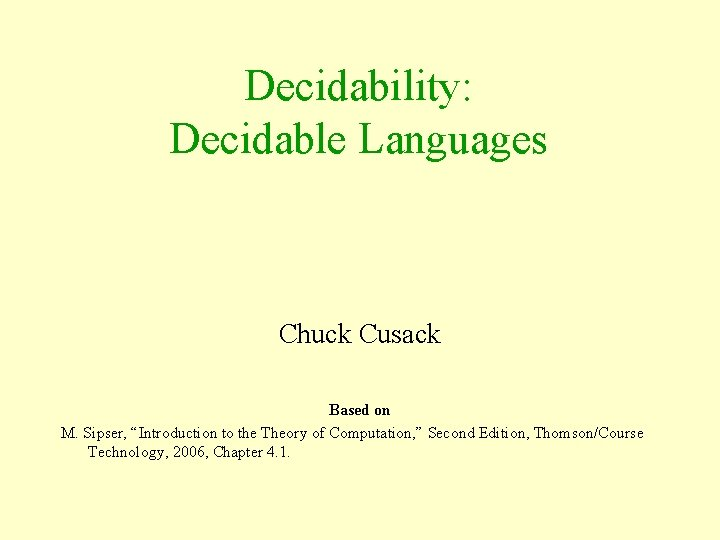Decidability: Decidable Languages Chuck Cusack Based on M. Sipser, “Introduction to the Theory of Computation, ” Second Edition, Thomson/Course Technology, 2006, Chapter 4. 1.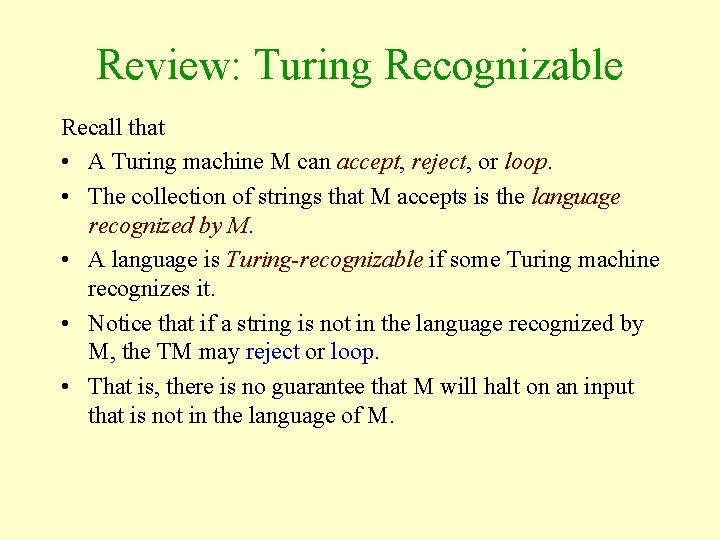Review: Turing Recognizable Recall that • A Turing machine M can accept, reject, or loop. • The collection of strings that M accepts is the language recognized by M. • A language is Turing-recognizable if some Turing machine recognizes it. • Notice that if a string is not in the language recognized by M, the TM may reject or loop. • That is, there is no guarantee that M will halt on an input that is not in the language of M.Review: Turing Decidable Recall that • A Turing machine M which always accepts of rejects (and never loops) is called a decider. • That is, a decider always returns an answer—“yes” or “no” —whereas a TM which is not a decider may loop on some inputs, never halting. • A decider that recognizes a language is said to decide the language. • A language is decidable if some Turing machine decides it.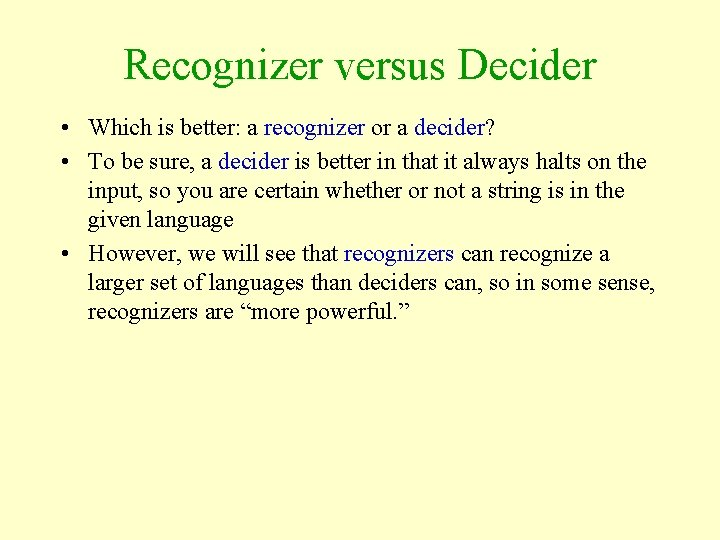Recognizer versus Decider • Which is better: a recognizer or a decider? • To be sure, a decider is better in that it always halts on the input, so you are certain whether or not a string is in the given language • However, we will see that recognizers can recognize a larger set of languages than deciders can, so in some sense, recognizers are “more powerful. ”Decidable Problems • Our next goal is to prove whether or not certain computational problems related to DFAs, CFGs, TMs, etc. , are solvable. • We are interested in – Acceptance Problem: Does a given “thing” accept/generate the given string? – Emptiness Testing: Does a given “thing” generate the empty language? – Equality Testing: Do two given “things” generate the same language?A Simple Example • Given a DFA A and a string w, I want to know whether or not A accepts w. • More generally, I want to know: – given any DFA and any input string, does the DFA generate the string? • We can represent this computational problem as the following language: ADFA = { B, w | B is a DFA that accepts w } • We can solve the computational problem iff we can test membership in the given language. • That is, the computational problem is solvable iff the language is decidable.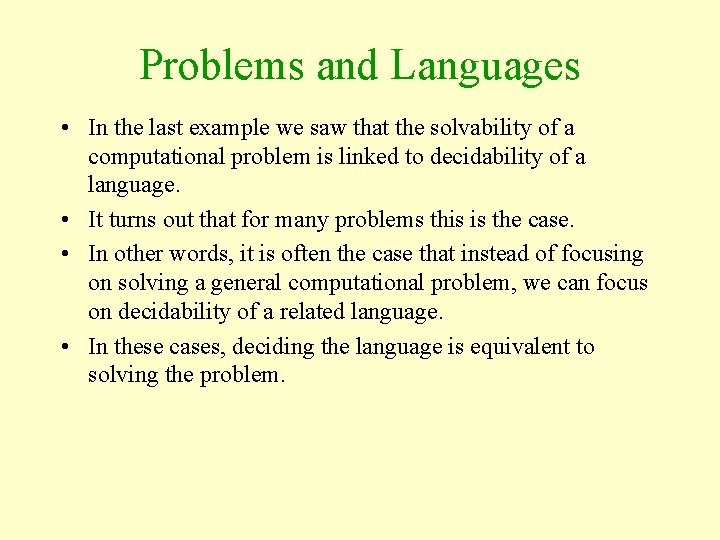Problems and Languages • In the last example we saw that the solvability of a computational problem is linked to decidability of a language. • It turns out that for many problems this is the case. • In other words, it is often the case that instead of focusing on solving a general computational problem, we can focus on decidability of a related language. • In these cases, deciding the language is equivalent to solving the problem.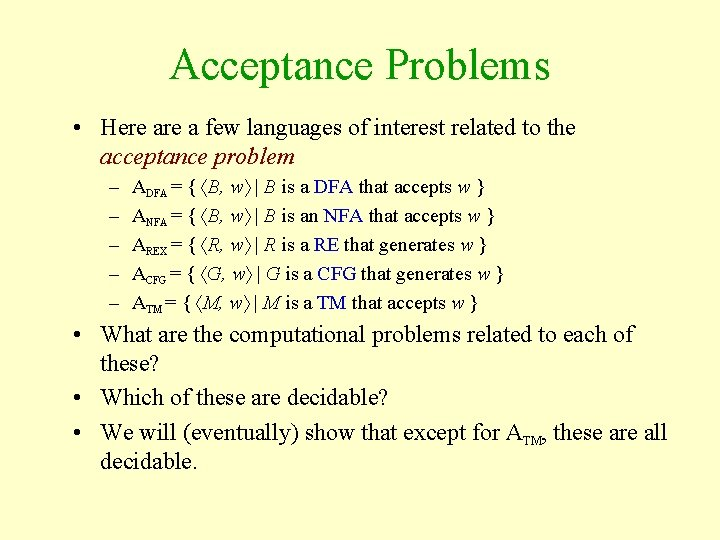Acceptance Problems • Here a few languages of interest related to the acceptance problem – – – ADFA = { B, w | B is a DFA that accepts w } ANFA = { B, w | B is an NFA that accepts w } AREX = { R, w | R is a RE that generates w } ACFG = { G, w | G is a CFG that generates w } ATM = { M, w | M is a TM that accepts w } • What are the computational problems related to each of these? • Which of these are decidable? • We will (eventually) show that except for ATM, these are all decidable.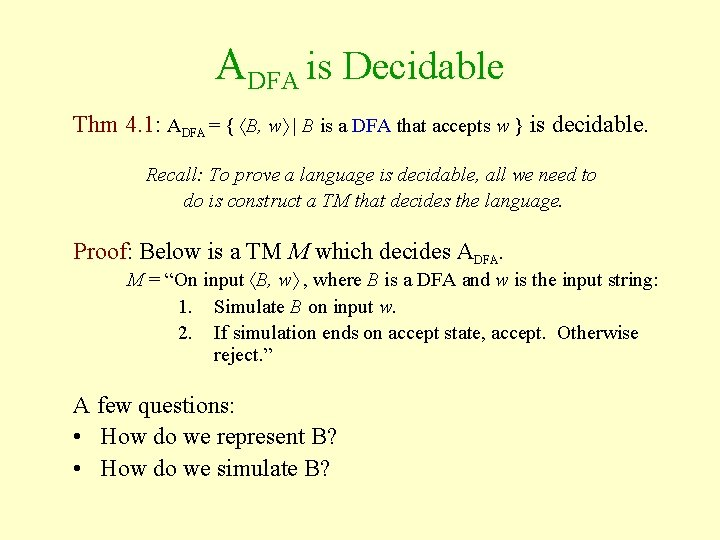ADFA is Decidable Thm 4. 1: ADFA = { B, w | B is a DFA that accepts w } is decidable. Recall: To prove a language is decidable, all we need to do is construct a TM that decides the language. Proof: Below is a TM M which decides ADFA. M = “On input B, w , where B is a DFA and w is the input string: 1. Simulate B on input w. 2. If simulation ends on accept state, accept. Otherwise reject. ” A few questions: • How do we represent B? • How do we simulate B?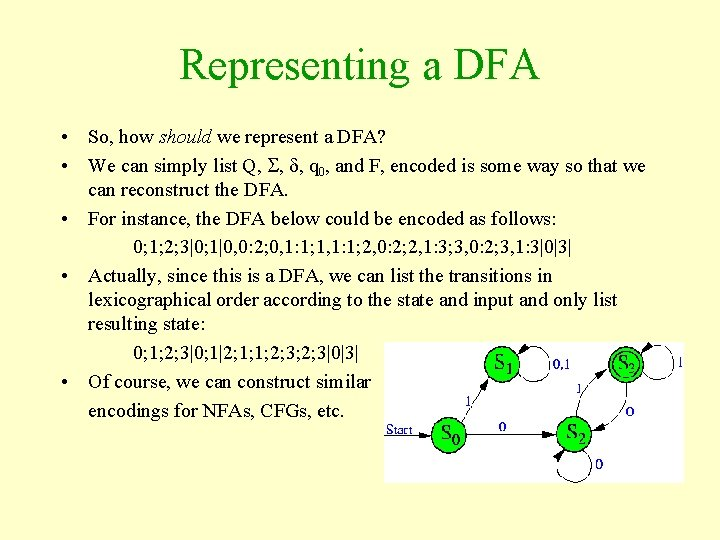Representing a DFA • So, how should we represent a DFA? • We can simply list Q, , , q 0, and F, encoded is some way so that we can reconstruct the DFA. • For instance, the DFA below could be encoded as follows: 0; 1; 2; 3|0; 1|0, 0: 2; 0, 1: 1; 1, 1: 1; 2, 0: 2; 2, 1: 3; 3, 0: 2; 3, 1: 3|0|3| • Actually, since this is a DFA, we can list the transitions in lexicographical order according to the state and input and only list resulting state: 0; 1; 2; 3|0; 1|2; 1; 1; 2; 3|0|3| • Of course, we can construct similar encodings for NFAs, CFGs, etc.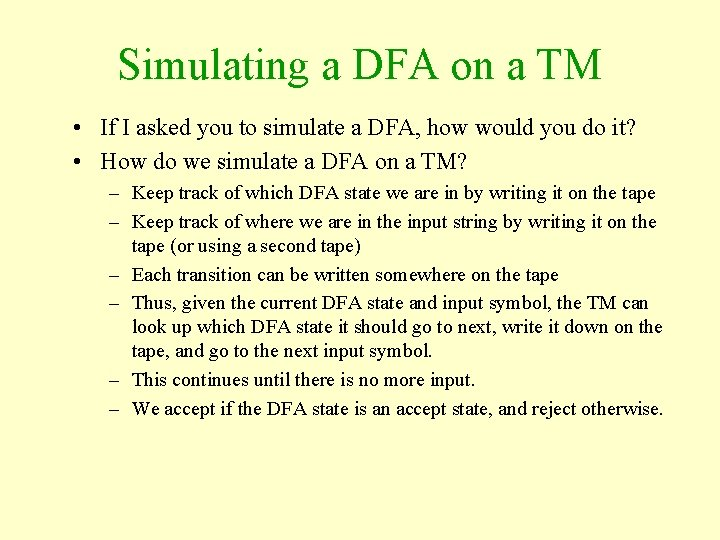Simulating a DFA on a TM • If I asked you to simulate a DFA, how would you do it? • How do we simulate a DFA on a TM? – Keep track of which DFA state we are in by writing it on the tape – Keep track of where we are in the input string by writing it on the tape (or using a second tape) – Each transition can be written somewhere on the tape – Thus, given the current DFA state and input symbol, the TM can look up which DFA state it should go to next, write it down on the tape, and go to the next input symbol. – This continues until there is no more input. – We accept if the DFA state is an accept state, and reject otherwise.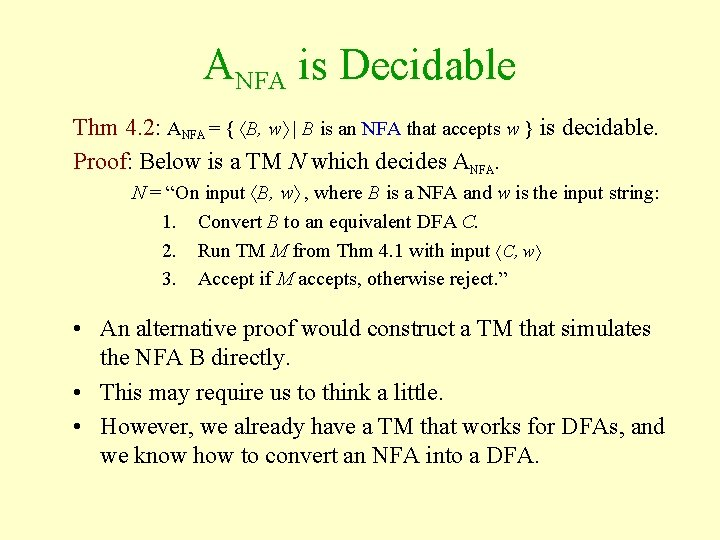ANFA is Decidable Thm 4. 2: ANFA = { B, w | B is an NFA that accepts w } is decidable. Proof: Below is a TM N which decides ANFA. N = “On input B, w , where B is a NFA and w is the input string: 1. Convert B to an equivalent DFA C. 2. Run TM M from Thm 4. 1 with input C, w 3. Accept if M accepts, otherwise reject. ” • An alternative proof would construct a TM that simulates the NFA B directly. • This may require us to think a little. • However, we already have a TM that works for DFAs, and we know how to convert an NFA into a DFA.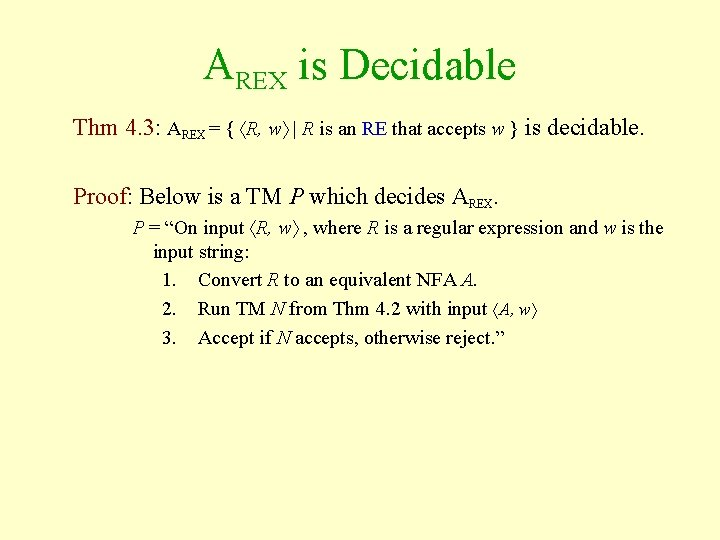AREX is Decidable Thm 4. 3: AREX = { R, w | R is an RE that accepts w } is decidable. Proof: Below is a TM P which decides AREX. P = “On input R, w , where R is a regular expression and w is the input string: 1. Convert R to an equivalent NFA A. 2. Run TM N from Thm 4. 2 with input A, w 3. Accept if N accepts, otherwise reject. ”The Problem of Emptiness • Languages related to the emptiness problem – EDFA = { B | B is a DFA and L(B) = } – ECFG = { G | G is a CFG and L(G) = } – ETM = { M | M is a TM and L(M) = } • What are the computational problems related to each of these? • Which of these are decidable? • Next we will show that EDFA is decidable • Later we will show that ECFG is decidable. • Eventually, we will show that ETM is not decidable.EDFA is Decidable Thm 4. 4: EDFA = { B | B is a DFA and L(B)= } is decidable. Proof: Below is a TM T which decides EDFA. T = “On input B , where B is a DFA: 1. Mark the start state of B. 2. Repeat until no new states are marked 3. Mark any state that has a transition to it from an already marked state. 4. If no accept states are marked, accept, otherwise reject. ” • The idea is that the language is empty only if there is no way to get to an accept state from the start state.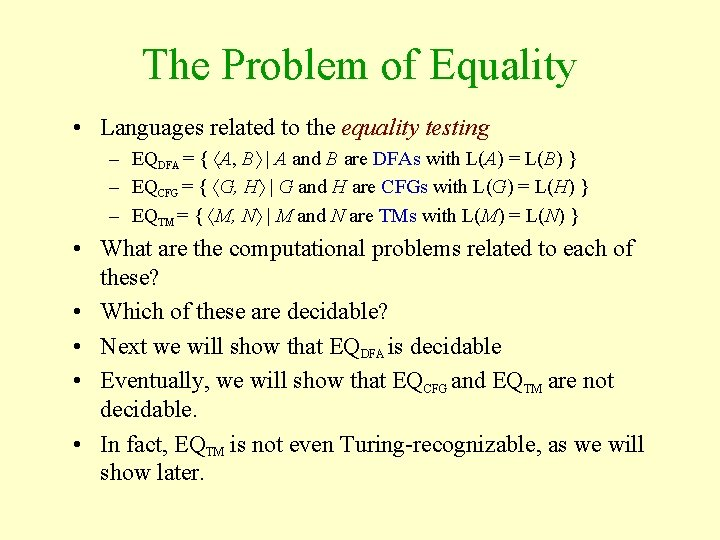The Problem of Equality • Languages related to the equality testing – EQDFA = { A, B | A and B are DFAs with L(A) = L(B) } – EQCFG = { G, H | G and H are CFGs with L(G) = L(H) } – EQTM = { M, N | M and N are TMs with L(M) = L(N) } • What are the computational problems related to each of these? • Which of these are decidable? • Next we will show that EQDFA is decidable • Eventually, we will show that EQCFG and EQTM are not decidable. • In fact, EQTM is not even Turing-recognizable, as we will show later.EQDFA is Decidable Thm 4. 5: EQDFA = { A, B | A and B are DFAs and L(A) = L(B) } is decidable. • Before we can prove this, we need a few observations – Let L(C) = (L(A) L(B) ) (L(A) L(B)) – L(C) is the symmetric difference of L(A) and L(B). – Notice that L(A) = L(B) iff L(C) = . – Thus, to test if L(A) = L(B), we can instead test if L(C) = . – Since L(C) is constructed using complements, intersections, and unions of regular sets, we can L(A) L(B) construct it with a TM. (Why? )EQDFA is Decidable (continued) Thm 4. 5: EQDFA = { A, B | A and B are DFAs and L(A) = L(B) } is decidable. Proof: Below is a TM F which decides EQDFA. T = “On input A, B , where A and B are DFAs: 1. Construct DFA C for language L(C). 2. Run TM T from Thm 4. 4 on input C. 3. Accept if T accepts, otherwise reject. ” We now move on to languages related to CFGs.ACFG is Decidable Thm 4. 7: ACFG = { G, w | G is a CFG that generates w } is decidable. Proof: Below is a TM S which decides ACFG S = “On input G, w , where G is a CFG and w is the input string: 1. Convert G to an equivalent CFG in CNF. 2. List all derivations which have 2 n-1 steps, where n = |w| (or 1 if |w| = 0). 3. Accept if any of the derivations generates, otherwise reject. ” • Why do we use CNF? • Why 2 n-1 steps? • Why not just use G and try derivations until we find one?ECFG is Decidable Thm 4. 8: ECFG = { G | G is a CFG and L(G)= } is decidable. Proof: Below is a TM R which decides ECFG. R = “On input G , where G is a CFG: 1. Mark all terminal symbols of G. 2. Repeat until no new variables are marked 3. Mark any variable A such that A→U 1 U 2…Uk is a rule and U 1, U 2, …Uk are all marked. 4. Accept if the start state is not marked, otherwise reject. ” • The only way the start state gets marked is if it is possible to apply rules in such a way that all variables are eventually replaced with terminal symbols.Is EQCFG Decidable? • Recall the languages – EQDFA = { A, B | A and B are DFAs with L(A) = L(B) } – EQCFG = { G, H | G and H are CFGs with L(G) = L(H) } • We proved that EQDFA was decidable by constructing a new language L(C) = (L(A) L(B) ) (L(A) L(B)) and proving that L(C) was empty, implying L(A) = L(B) • Can we use this same technique to show that EQCFG? • Recall that CFGs are not closed under intersection or complement, so this idea won’t work. • In fact, you cannot prove that EQCFG is decidable, because it is not! • We will prove this fact later.Is every CFL Decidable? • Given a context free language A, we would like to be able to determine whether or not a string is in that language. • That is, we want to know that for any given CFL A, A is decidable. • If we could show this, then we could draw this relationship between classes of languages: • Luckily for us, we can Turingshow this, which we recognizable will do next. Decidable • Thus, the diagram to the Context-free right is correct. RegularEvery CFL is Decidable Thm 4. 9: Every CFL is decidable. Proof: Let A be a CFL. Given any string w, we need to determine whether or not w is in A. Let G be a CFG generating A. The TM MG below decides A. MG = “On input w 1. Run TM S from Thm 4. 7 on input G, w. 2. Accept if S accepts, otherwise reject. ”